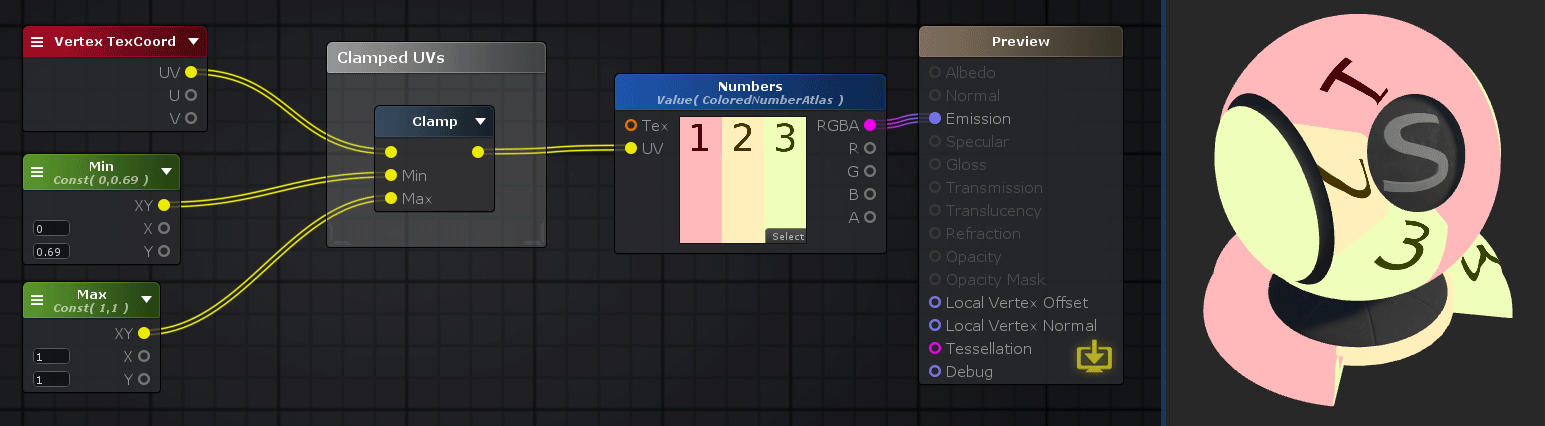## Clamp Node

The Clamp node outputs its Input value or individual components of vectors clamped between the [ Min , Max ] range.

• Min: This value is returned if input value is less than Min
• Input: This value is returned if input value is between Min and Max
• Max: This value is returned if input value greater than Max

NOTE: If each input port have different channel amounts a cast is done to match the one with the most amount of channels.Nodes used: Vertex TexCoord, Float, Clamp, Texture Sample

Node Parameter Description Default Value
Input Value to be clamped between the [ Min , Max ] range. Only visible if the respective input port is not connected. 0
Min Minimum value of the clamp operation, if value is less than Min than this is the returned value. Only visible if the respective input port is not connected. 0
Max Maximum value of the clamp operation, if value is greater than Max than this is the returned value. Only visible if the respective input port is not connected. 1

Input Port Description Type
Input Value to be clamped between the [ Min , Max ] range. Fully outputted when Alpha = 0. Float 
Min Minimum value of the clamp operation, if value is less than Min than this is the returned value. Float 
Max Maximum value of the clamp operation, if value is greater than Max than this is the returned value. Float 

1. ^ Port automatically adapts to all connection types except Matrices and Sampler types.

 Math Operators - Max/Min/Clamp